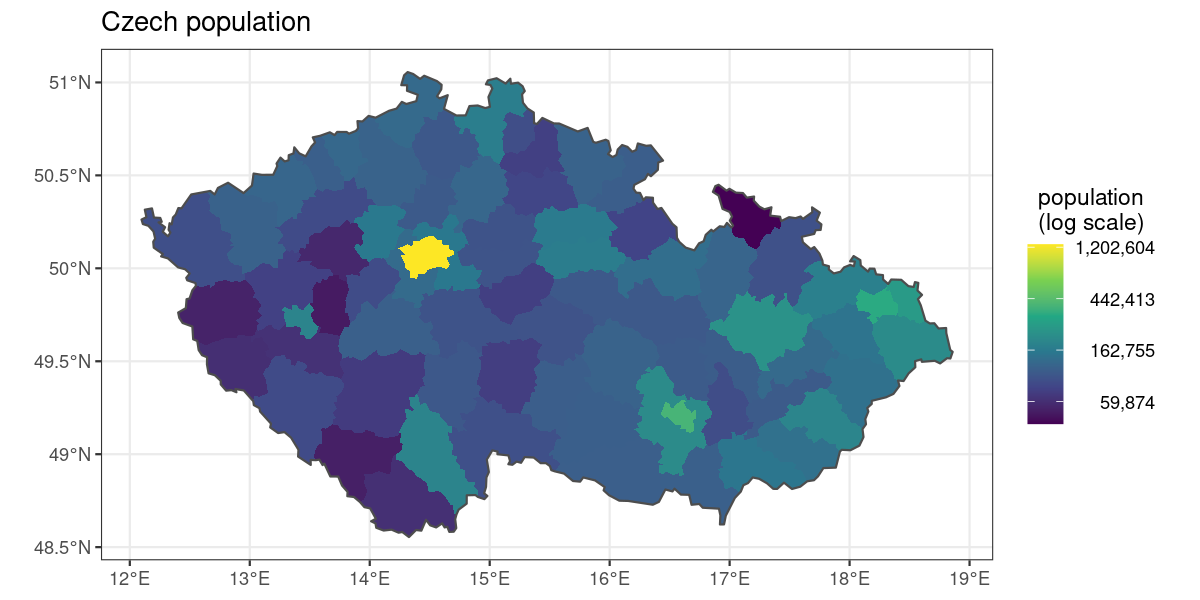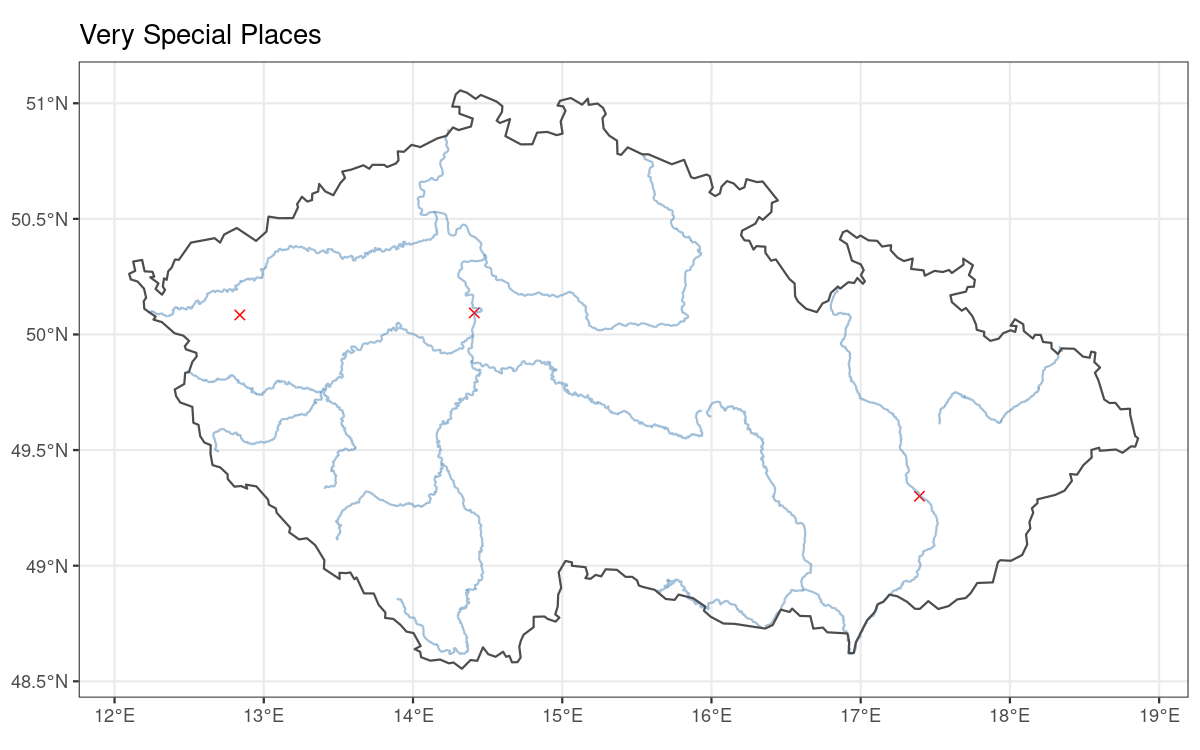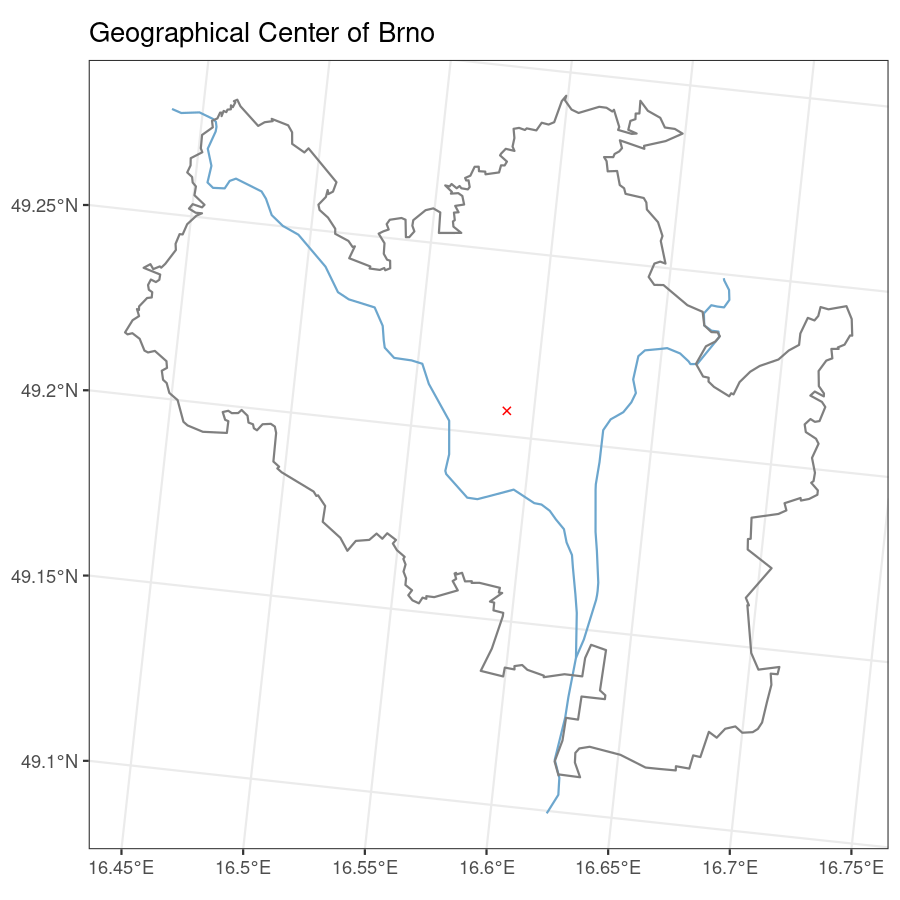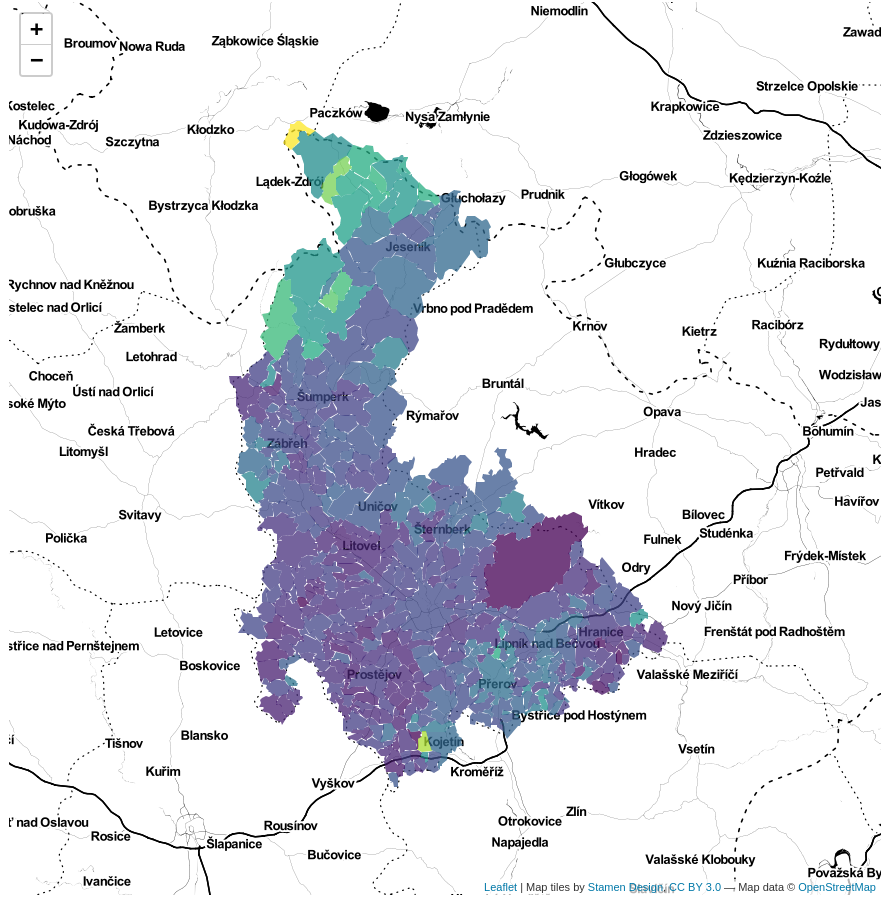# Solving Real World Issues With RCzechia

### Visualizing Czech Population

Population of the Czech Republic as per the latest census in 2011, per district (okres).

As the population distributed unevenly a log scale is used.

``````library(RCzechia)
library(ggplot2)
library(dplyr)
library(httr)
library(sf)

tf <- tempfile(fileext = ".xls") # a temporary xls file

GET("https://raw.githubusercontent.com/jlacko/RCzechia/master/data-raw/zvcr034.xls",
write_disk(tf))
## Response [https://raw.githubusercontent.com/jlacko/RCzechia/master/data-raw/zvcr034.xls]
##   Date: 2020-05-04 11:05
##   Status: 200
##   Content-Type: application/octet-stream
##   Size: 44.5 kB
## <ON DISK>  /tmp/RtmptlIfQ1/file16e026105a8.xls

src <- read_excel(tf, range = "Data!B5:C97") # read in with original column names

colnames(src) <- c("NAZ_LAU1", "obyvatel") # meaningful names instead of the original ones

src <- src %>%
mutate(obyvatel = as.double(obyvatel)) %>%
# convert from text to number
mutate(NAZ_LAU1 = ifelse(NAZ_LAU1 == "Hlavní město Praha", "Praha", NAZ_LAU1))
# rename Prague (from The Capital to a regular city)

okresni_data <- RCzechia::okresy("low") %>% # data shapefile
inner_join(src, by = "NAZ_LAU1")
# key for data connection - note the use of inner (i.e. filtering) join

# report results
ggplot(data = okresni_data) +
geom_sf(aes(fill = obyvatel), colour = NA) +
geom_sf(data = republika("low"), color = "gray30", fill = NA) +
scale_fill_viridis_c(trans = "log", labels = scales::comma) +
labs(title = "Czech population",
fill = "population\n(log scale)") +
theme_bw() +
theme(legend.text.align = 1,
legend.title.align = 0.5)``````## Geocoding Locations & Drawing them on a Map

Drawing a map: three semi-random landmarks on map, with rivers shown for better orientation.

To get the geocoded data frame function `RCzechia::geocode()` is used.

``````library(RCzechia)
library(ggplot2)
library(sf)

borders <- RCzechia::republika("low")

rivers <- subset(RCzechia::reky(), Major == T)

mista <- data.frame(misto =  c("Kramářova vila",
adresa = c("Gogolova 212, Praha 1",
"Sněmovní náměstí 1, Kroměříž",
"nám. 5. května 1, Bečov nad Teplou"))

# from a string vector to sf spatial points object

class(POI) # in {sf} package format = spatial and data frame
##  "sf"         "data.frame"

# report results
ggplot() +
geom_sf(data = POI, color = "red", shape = 4, size = 2) +
geom_sf(data = rivers, color = "steelblue", alpha = 0.5) +
geom_sf(data = borders, color = "grey30", fill = NA) +
labs(title = "Very Special Places") +
theme_bw()``````## Distance Between Prague and Brno

Calculate distance between two spatial objects; the `sf` package supports (via gdal) point to point, point to polygon and polygon to polygon distances.

Calculating distance from Prague (#1 Czech city) to Brno (#2 Czech city).

``````library(dplyr)
library(RCzechia)
library(sf)
library(units)

obce <- RCzechia::obce_polygony()

praha <- subset(obce, NAZ_OBEC == "Praha")

brno <- subset(obce, NAZ_OBEC == "Brno")

vzdalenost <- sf::st_distance(praha, brno) %>%
units::set_units("kilometers") # easier to interpret than meters, miles or decimal degrees..

# report results
print(vzdalenost)
## 152.8073 [kilometers]``````

## Geographical Center of the City of Brno

The metaphysical center of the Brno City is well known. But where is the geographical center?

The center is calculated using `sf::st_centroid()` and reversely geocoded via `RCzechia::revgeo()`.

Note the use of `reky("Brno")` to provide the parts of Svitava and Svratka relevant to a map of Brno city.

``````library(dplyr)
library(RCzechia)
library(ggplot2)
library(sf)

# polygon of Brno city
brno <- dplyr::filter(RCzechia::okresy(), KOD_LAU1 == "CZ0642")

# calculate centroid
pupek_brna <- brno %>%
st_transform(5514) %>% # planar CRS (eastings & northings)
st_set_agr('constant') %>%  # not strictly necessary, but avoids error message
sf::st_centroid(brno) # calculate central point of a polygon

# the revgeo() function takes a sf points data frame and returns it back
# with address data in "revgeocoded"" column

# report results
##  "Žižkova 513/22, Veveří, 61600 Brno"

ggplot() +
geom_sf(data = pupek_brna, col = "red", shape = 4) +
geom_sf(data = reky("Brno"), color = "skyblue3") +
geom_sf(data = brno, color = "grey50", fill = NA) +
labs(title = "Geographical Center of Brno") +
theme_bw()``````## Interactive Map

Interactive maps are powerful tools for data visualization. They are easy to produce with the `leaflet` package.

I found the stamen toner basemap a good company for interactive chloropleths - it gives enough context without distracting from the story of your data.

Note: it is technically impossible to make html in vignette interactive. As a consequence the result of code shown has been replaced by a static screenshot; the code itself is legit.

``````library(dplyr)
library(RCzechia)
library(leaflet)
library(sf)

src <- read.csv(url("https://raw.githubusercontent.com/jlacko/RCzechia/master/data-raw/unempl.csv"), stringsAsFactors = F)
# open data on unemployment from Czech Statistical Office - https://www.czso.cz/csu/czso/otevrena_data
# lightly edited for size (rows filtered)

src <- src %>%
mutate(KOD_OBEC = as.character(uzemi_kod))  # keys in RCzechia are of type character

podklad <- RCzechia::obce_polygony() %>% # obce_polygony = municipalities in RCzechia package
inner_join(src, by = "KOD_OBEC") %>% # linking by key
filter(KOD_CZNUTS3 == "CZ071") # Olomoucký kraj

pal <- colorNumeric(palette = "viridis",  domain = podklad\$hodnota)

leaflet() %>%
fillColor = ~pal(hodnota),
fillOpacity = 0.75,
color = NA)``````## Dissolving `sf` Polygons

Creating custom polygons by aggregating administrative units is a common use case in sales reporting and analysis.

In many use cases a simple `dplyr::group_by() %>% dplyr::summarize()` call will do. Some shapefiles (unfortunately including the ArcČR®500, on which `{RCzechia}` is based) are not that well behaved, and require repairing of faulty geometry.

Function `RCzechia::union_sf()` makes this task easier by automating some of the more common polygon repair tricks.

In this demonstration the Czech LAU1 units are grouped into two categories: those with odd lettered names, and those with even letters. They are then dissolved into two multipolygons.

``````library(RCzechia)
library(ggplot2)
library(dplyr)
library(sf)

poly <- RCzechia::okresy("low") %>% # Czech LAU1 regions as sf data frame
mutate(oddeven = ifelse(nchar(NAZ_LAU1) %% 2 == 1, "odd", "even" )) %>% # odd or even?
RCzechia::union_sf("oddeven") # ... et facta est lux

# Structure of the "poly" object:
## Simple feature collection with 2 features and 1 field
## geometry type:  MULTIPOLYGON
## dimension:      XY
## bbox:           xmin: 12.09771 ymin: 48.55407 xmax: 18.85924 ymax: 51.05574
## geographic CRS: WGS 84
##   oddeven                       geometry
## 1     odd MULTIPOLYGON (((14.73059 50...
## 2    even MULTIPOLYGON (((16.75784 49...

# report results
ggplot(data = poly, aes(fill = oddeven)) +
geom_sf() +
scale_fill_viridis_d() +
labs(title = "Number of characters in names of Czech districts",
fill = "Odd or even?") +
theme_bw()``````